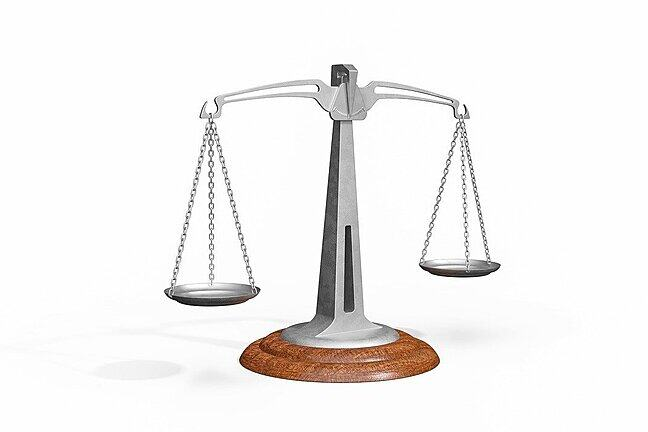## Want to keep learning?

This content is taken from the University of York's online course, Logic: The Language of Truth. Join the course to learn more.
3.22

## University of York# Logical equivalence

We can approach the concept of logical equivalence by first looking at a sentence connective in English that isn’t one of our main targets.

Consider

• Neither kale nor spinach are fun to eat

As it stands, this doesn’t obviously have the right kind of structure to be treated in terms of sentence connectives: ‘kale’ isn’t a sentence. We can fix this with a paraphrase:

• Neither is it the case that kale is fun to eat nor it is the case that spinach is fun to eat

But now we might ask what’s the meaning of ‘Neither is it the case that … nor it is the case that …’. Is it a truth-functional sentence connective? If it is, what are its truth-conditions?

Suppose we define basic sentence letters as follows:

• K: Kale is fun to eat
• S: Spinach is fun to eat

The connective seems to be related to ‘or’, but has a ‘not-ish’ flavour. These hints might make us think we might try combining vel and tilde to capture its content. Perhaps something like:

• ~(K $$\vee$$ S)

But suppose that, instead of just following hints from similarities between words, we adopt a more hard-headed approach and think about the circumstances under which ‘Neither is it the case that kale is fun to eat nor it is the case that spinach is fun to eat’ would be true. It seems like it’s true only where ‘K’ and ‘S’ are both false—that is, where:

• (~K & ~S)

Which is right?

Let’s look at their truth-tables. (We’ve omitted the columns for the basic sentences from this table so we can show the results clearly. You’ll see from the values for the basic sentences that it’s arranged in the standard way.)

~ (K $$\vee$$ S )   (~ K & ~ S)
F t T t   F t F F t
F t T f   F t F T f
F f T t   T f F F t
T f F f   T f T T f

This table shows our two candidates are true in exactly the same circumstances (and false in exactly the same circumstances). There is only one kind of situation in which they’re true: where ‘K’ and ‘S’ are both false. Otherwise they’re false. When two sentences are true in exactly the same circumstances (and false in exactly the same circumstances) we say they are logically equivalent.

So, both of our candidates are right (if we’re happy that these are the truth-conditions of the original sentence).

The term ‘logically equivalent’ applies because they’re going to be exactly alike in terms of what entails them and what (in combination with other claims) they entail: from the point of view of the logic of deductive validity, they have exactly matched powers.

(You can find videos showing the use of truth-tables to demonstrate further cases of logical equivalence in two steps in Week 4’s materials.)